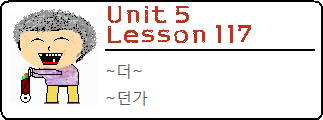Lesson 117: In this lesson, you will be introduced to the grammatical principle ~더~ and the meaning it creates in a sentence. You will apply your knowledge of –더- by combining it with another grammatical principle to create ~던가.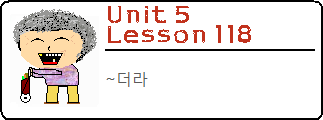Lesson 118: In this lesson, you will continue to learn about ~더~. Specifically, you will learn about ~더라~, which is a common ending to a sentence.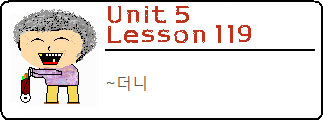Lesson 119: In this lesson, we will finish with our group of lessons about ~더~. Specifically, in this lesson, you will learn how to use ~더~ in combination with ~(으)니 (Lesson 81) to connect two clauses. The construction ~더니 is very similar to other clause connectors like ~아/어서, ~(으)니(까) and ~기 때문에, but as always there are some specific usages you should know about.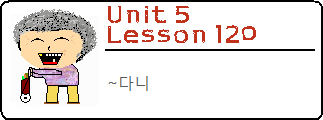Lesson 120: In this lesson, you will learn how to connect two clauses with ~다니, which will be your first real introduction into the world of compounded quoted grammatical principles – which is a term I just made up off the top of my head.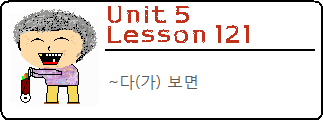Lesson 121: In this lesson, you will learn how to connect two clauses by using the grammatical principle ~다 보면. The grammar within this principle is actually the addition of a few different grammar concepts piled together, but its meaning is simple enough to describe in a short lesson.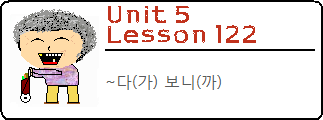Lesson 122: In this lesson, you will learn another grammatical principle that requires the addition of “보다” between two clauses. Specifically, you will learn how to use ~다 보니 to connect two clauses.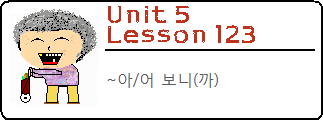Lesson 123: In this lesson, you will continue to learn about grammatical principles that contain “보다”. In this lesson, you will specifically learn about the grammatical principle: ~아/어 보니까.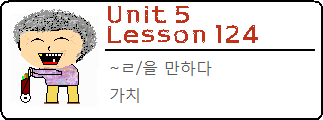Lesson 124: In this lesson, you will learn how to use the grammatical principle ~ㄹ/을 만하다 to indicate that something is worth doing or possible. In doing so, you will see how these types of sentences can also be expressed using the word 가치.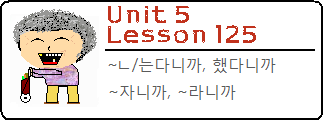Lesson 125: In this lesson, you will learn a Korean grammatical principle that you can use to make your Korean sound extra sassy! You can use ~ㄴ/는다니까 when you are a little bit annoyed at somebody, and what to show your frustration.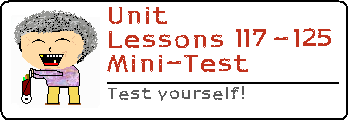Lessons 117 – 125 Mini-Test: Test yourself on what you learned from Lessons 117 to 125!